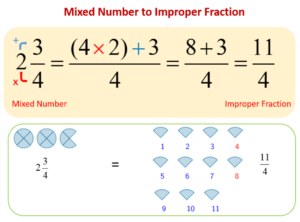# MS Math – October 18-226th Grade Math – Mrs. Evans

This week we will be dividing fractions and mixed numbers.  Dividing fractions is actually a pretty simple thing to do, especially after we know how to multiply fractions.  This is because a division can be considered the same as multiplying by the reciprocal.  A reciprocal is just the “flip” of a number or fraction.  For example the reciprocal of 3/5 is 5/3.  The numerators and denominators just trade places.  We have a great way to remember how to divide by fractions:When dividing with a mixed number, just as we do in multiplying, we switch the mixed number into an improper fraction and then continue as if it were any other fraction.  Here is a reminder for those who need help converting a mixed number into an improper fraction:We will practice being able to apply our new skills in problem solving as we collaborate with each other.  We will also focus on being able to verbalize how to multiply and divide fractions.  We will finish the week with a KAHOOT covering all the topics from Chapter 2.

Math Grade Math – Mrs. Evans and Mrs. VonFeldt

This week we will continue working in Module 3, not only adding and subtracting positive and negative rational numbers but multiplying and dividing them.  These are foundational skills requiring a degree of fluency.  Students who are struggling with these skills should seek help so that they are confident as the move into algebra later in the year.  A great place for extra help with immediate feedback on how a student is doing, is http://IXL.COM

The rules we learned with integers that help us to multiply and divide are still applicable with rational numbers.  Here are the rules to remind us:8th Grade Math – Mrs. Ernest

This week students will be refining their graphing skills, practice finding the difference between points, and interpret graphs through word problems. Students struggling with graphing skills should revisit these IXL activities: